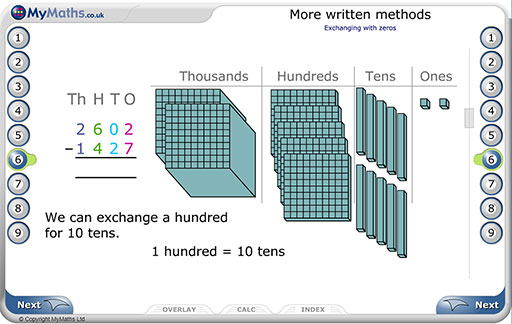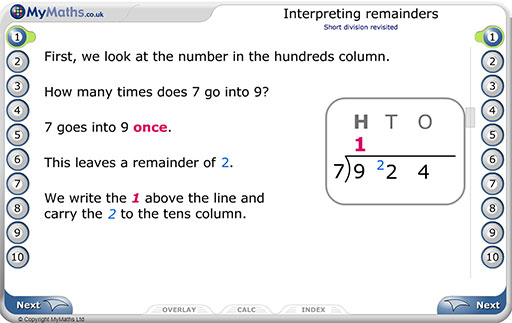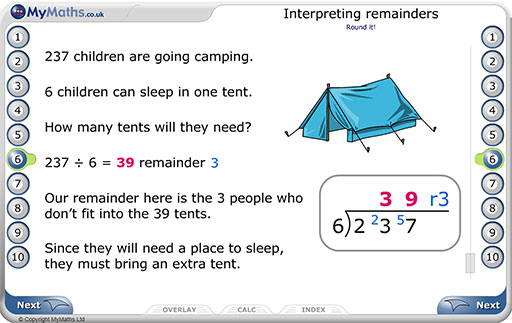With SATs just around the corner, it’s a great time of year to brush up on some core skills like formal written methods.

MyMaths Editor Kristie takes a closer look at some of the MyMaths resources that could help your students to hone their skills.

## More written methods

This lesson begins by recapping column addition with 3-digit numbers, before progressing to examples with 4-digit numbers. Each page of teaching is followed by a ‘Your turn’ page, which gives children a chance to put what they have just seen into action.

The lesson then moves on to subtraction, focussing on tricky examples involving exchanging. The clear step-by step examples are accompanied by base 10 apparatus, so that children have concrete understanding of the procedure.Each page of teaching is followed by a ‘Your turn’ page, which gives children a chance to put what they have just seen into action.The clear step-by step examples are accompanied by base 10 apparatus, so that children have concrete understanding of the procedure.

Finally, the lesson delves into the important skill of estimation, and how we can use this to check our calculations for mistakes. The final word problem brings all the skills covered in the lesson together, and ensures children are able to apply them in context.

By setting the matching homework activity, you can find out which children have a strong grasp of written methods of addition and subtraction. If they have found the homework difficult, you may want to encourage children to work through the ‘Introducing column addition’ and ‘Introducing column subtraction’ lesson and homework pairs, to secure the basics. If they found it straightforward, you could encourage them to work through the ‘Addition and subtraction problems’ lesson and homework, which includes a collection of contextual problems that require a variety of mental and written strategies to solve.

Find this lesson on MyMaths by searching for “More written methods” in the Library.

## Interpreting remainders

This lesson recaps the method of short division with 3-digit numbers, before progressing on to division with 4-digit numbers. Both are shown through easy to follow, step-by-step examples.We consider questions where the remainder can be ignored, where it can be rounded, and where it can be presented as a fraction or decimal.

The lesson then explores divisions with remainders, looking at the ways we may deal with a remainder, depending on the context of a question. We consider questions where the remainder can be ignored, where it can be rounded, and where it can be presented as a fraction or decimal.Find this lesson on MyMaths by searching for “Interpreting remainders” in the Library.

Considering rich questions like these is great practice for the reasoning paper, where children will need to not only grasp the formal methods, but be able to modify their answer according to the context.

## Short and Long multiplication

This lesson reminds children of the short multiplication method, before delving into long multiplication. Scaffolded questions guide children through every step of the method.

Throughout the lesson there are lots of word problems, to make sure children are comfortable applying their skills in context.